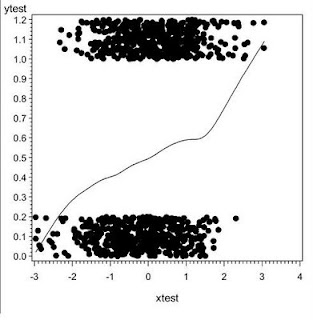## Wednesday, June 24, 2009

### Example 7.3: Simple jittered scatterplot with smoother for dichotomous outcomes with continuous predictors

It's useful to look at scatterplots even when the "y" variable is dichotomous. For example, this can help determine whether categorization or linear assumptions would be more plausible. However, an unmodified scatterplot is less than helpful, since all of the "y" values are either 0 or 1, and are hard to separate visually. Some jittering (section 5.2.4) is useful in that regard. In addition, it is often useful to plot a smoothed line through the data. We use the data generated in section 7.2 to demonstrate.

SAS
In SAS, we add jitter, then plot the jittered values and the observed values on the same plot using the overlay option. We display the jittered values as dots and add a smoothed line through the real (not jittered) data without displaying their values using symbol statements (sections 5.2.2, 5.2.6).
`data ds2;set test;yplot = ytest + uniform(0) * .2;run;symbol1 i = sm50s v = none c = black;symbol2 i = none v = dot c = black;proc gplot data = ds2;plot (ytest yplot) * xtest / overlay;run;`
And the resulting plot is:
``

R
In R, we display a scatterplot (section 5.1.1) of the jittered values against the covariate. The jitter() function (section 5.2.4) is called within the plot() function. We then add the smoothed line, based on the real (not jittered) data using the lines() function (section 5.2.1), called with the appropriate lowess() (section 5.2.6) object as input.
`plot(xtest,jitter(ytest))lines(lowess(xtest,ytest))`

And the resulting plot is:These plots are useful, but fairly unattractive. In our next example, we'll make them prettier.

#### 1 comment:

Ken Kleinman said...

A reader asked why the two smoothed lines look so different. Two reasons: first, they're using different smoothers, and second, they use the simulated data generated in Example 7.2, which are different between the two programs.